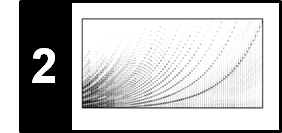# STFT: Frequency Grid Density

Computing a discrete STFT introduces a frequency grid which resolution depends on the signal's sampling rate and the STFT window size, see Section 2.1.4 of [Müller, FMP, Springer 2015]. In this notebook, we discuss how to make the frequency grid denser by suitably padding the windowed sections in the STFT computation. Often, one loosely says that this procedure increases the frequency resolution. This, however, is not true in a qualitative sense as we explain below.

## DFT Frequency Grid¶

Let $x\in \mathbb{R}^N$ be discrete signal of length $N\in\mathbb{N}$ with samples $x(0), x(1), \ldots, x(N-1)$. Given the sampling rate $F_\mathrm{s}$, we assume that $x$ is obtained by sampling a continues-time signal $f:\mathbb{R}\to\mathbb{R}$. Then, the discrete Fourier transform (DFT) $X := \mathrm{DFT}_N \cdot x$ can be interpreted as an approximation of the continuous Fourier transform $\hat{f}$ for certain frequencies (see Equation (2.132) of [Müller, FMP, Springer 2015]):

$$X(k) := \sum_{n=0}^{N-1} x(n) \exp(-2 \pi i k n / N) \approx {F_\mathrm{s}} \cdot \hat{f} \left(k \cdot \frac{F_\mathrm{s}}{N}\right)$$

for $k\in[0:N-1]$. Thus, the index $k$ of $X(k)$ corresponds to the physical frequency

\begin{equation} F_\mathrm{coef}^N(k) := \frac{k\cdot F_\mathrm{s}}{N} \end{equation}

given in Hertz. In other words, the discrete Fourier transform introduces a linear frequency grid of resolution $F_\mathrm{s}/N$, which depends on the size $N$ of the $\mathrm{DFT}_N$. To increase the density of the frequency grid, one idea is to increase the size of the DFT by artificially appending zeros to the signal. To this end, let $L\in\mathbb{N}$ with $L\geq N$. Then, we apply zero padding to the right of the signal $x$ obtaining the signal $\tilde{x}\in \mathbb{R}^L$:

\begin{equation} \tilde{x}(n) :=\left\{\begin{array}{ll} x(n) ,& \,\,\mbox{for}\,\, n \in[0:N-1],\\ 0, & \,\,\mbox{for}\,\, n \in[N:L-1]. \end{array}\right. \end{equation}

Applying a $\mathrm{DFT}_L$, we obtain:

$$\tilde{X}(k) = \mathrm{DFT}_L \cdot \tilde{x} = \sum_{n=0}^{L-1} \tilde{x}(n) \exp(-2 \pi i k n / L) = \sum_{n=0}^{N-1} x(n) \exp(-2 \pi i k n / L) \approx {F_\mathrm{s}} \cdot \hat{f} \left(k \cdot \frac{F_\mathrm{s}}{L}\right)$$

for $k\in[0:L-1]$. The coefficient $\tilde{X}(k)$ now corresponds to the physical frequency

\begin{equation} F_\mathrm{coef}^L(k) := \frac{k\cdot F_\mathrm{s}}{L}, \end{equation}

thus introducing a linear frequency resolution of $F_\mathrm{s}/L$. For example, if $L=2N$, the frequency grid resolution is increased by a factor of $2$. In other words, the longer DFT results in more frequency bins that are more closely spaced. However, note that this trick does not improve the approximation quality of the DFT (note that the number of summands in the Riemann approximation is still $N$). Only, the linear sampling of the frequency axis is refined when using $L\geq N$ and zero padding. The following example compares $\mathrm{DFT}_N \cdot x$ with $\mathrm{DFT}_L \cdot \tilde{x}$.

In :
import numpy as np
from  matplotlib import pyplot as plt
import librosa
%matplotlib inline

Fs = 32
duration = 2
freq1 = 5
freq2 = 15
N = int(duration * Fs)
t = np.arange(N) / Fs
t1 = t[:N//2]
t2 = t[N//2:]

x1 = 1.0 * np.sin(2 * np.pi * freq1 * t1)
x2 = 0.7 * np.sin(2 * np.pi * freq2 * t2)
x = np.concatenate((x1, x2))

plt.figure(figsize=(8, 2))

ax1 = plt.subplot(1, 2, 1)
plt.plot(x, c='k')
plt.title('Orginal signal ($N$=%d)' % N)
plt.xlabel('Time (samples)')
plt.xlim([0, N - 1])
plt.subplot(1, 2, 2)
Y = np.abs(np.fft.fft(x)) / Fs
plt.plot(Y, c='k')
plt.title('Magnitude DFT of original signal ($N$=%d)' % N)
plt.xlabel('Frequency (bins)')
plt.xlim([0, N - 1])
plt.tight_layout()

L = 2 * N
t_tilde = np.concatenate((t, np.arange(len(x), len(x) + pad_len) / Fs))

plt.figure(figsize=(8, 2))
ax1 = plt.subplot(1, 2, 1)
plt.plot(x_tilde, c='k')
plt.title('Padded signal ($L$=%d)' % L)
plt.xlabel('Time (samples)')
plt.xlim([0, L - 1])
plt.subplot(1, 2, 2)
Y_tilde = np.abs(np.fft.fft(x_tilde)) / Fs
plt.plot(Y_tilde, c='k')
plt.title('Magnitude DFT of padded signal ($L$=%d)' % L)
plt.xlabel('Frequency (bins)')
plt.xlim([0, L - 1])

plt.tight_layout()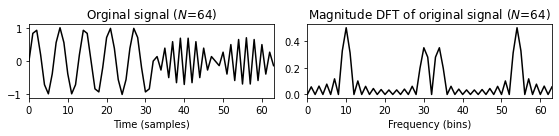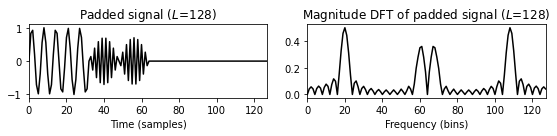The next code example implements a function for computing the DFT with increased frequency grid resolution, where all parameters are interpreted in a physical way (in terms of seconds and Hertz).

In :
def compute_plot_DFT_extended(t, x, Fs, L):
N = len(x)
t_tilde = np.concatenate((t, np.arange(len(x), len(x) + pad_len) / Fs))
Y = np.abs(np.fft.fft(x_tilde)) / Fs
Y = Y[:L//2]
freq = np.arange(L//2)*Fs/L
# freq = np.fft.fftfreq(L, d=1/Fs)
# freq = freq[:L//2]
plt.figure(figsize=(12, 2))

ax1 = plt.subplot(1, 3, 1)
plt.plot(t_tilde, x_tilde, c='k')
plt.title('Signal ($N$=%d)' % N)
plt.xlabel('Time (seconds)')
plt.xlim([t, t[-1]])

ax2 = plt.subplot(1, 3, 2)
plt.plot(t_tilde, x_tilde, c='k')
plt.title('Padded signal (of size $L$=%d)' % L)
plt.xlabel('Time (seconds)')
plt.xlim([t_tilde, t_tilde[-1]])

ax3 = plt.subplot(1, 3, 3)
plt.plot(freq, Y, c='k')
plt.title('Magnitude DFT of padded signal ($L$=%d)' % L)
plt.xlabel('Frequency (Hz)')
plt.xlim([freq, freq[-1]])
plt.tight_layout()

return ax1, ax2, ax3

N = len(x)

L = N
ax1, ax2, ax3 = compute_plot_DFT_extended(t, x, Fs, L)

L = 2 * N
ax1, ax2, ax3 = compute_plot_DFT_extended(t, x, Fs, L)

L = 4 * N
ax1, ax2, ax3 = compute_plot_DFT_extended(t, x, Fs, L)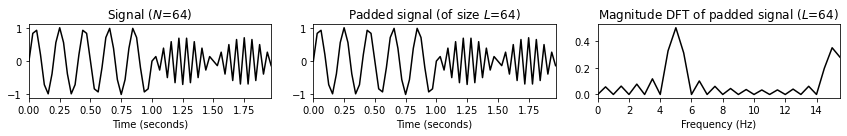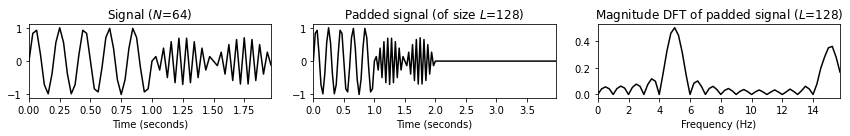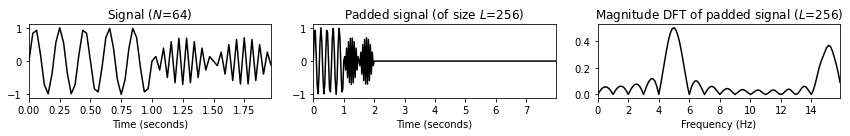## STFT with Increased Frequency Grid Resolution¶

We now show how the same zero-padding strategy can be used to increase the frequency grid resolution of an STFT. The librosa function librosa.stft implements this idea by means of the two parameters n_fft (corresponding to $L$) and win_length (corresponding to $N$). Care has to be taken when converting the parameters to the physical domain. Our example is the note C4 played by a violin (with vibrato).

In :
import os
import IPython.display as ipd

fn_wav = os.path.join('..', 'data', 'C2', 'FMP_C2_F05c_C4_violin.wav')

Fs = 11025
ipd.display(ipd.Audio(x, rate=Fs))

t_wav = np.arange(0, x.shape) * 1 / Fs
plt.figure(figsize=(5, 1.5))
plt.plot(t_wav, x, c='gray')
plt.xlim([t_wav, t_wav[-1]])
plt.xlabel('Time (seconds)')
plt.tight_layout()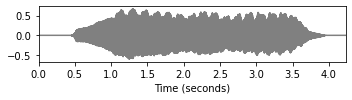We now compute an STFT with zero-padding. In the visualization, the axis are shown in terms of time frames and frequency bins.

In :
def compute_stft(x, Fs, N, H, L=N, pad_mode='constant', center=True):
X = librosa.stft(x, n_fft=L, hop_length=H, win_length=N,
Y = np.log(1 + 100 * np.abs(X) ** 2)
F_coef = librosa.fft_frequencies(sr=Fs, n_fft=L)
T_coef = librosa.frames_to_time(np.arange(X.shape), sr=Fs, hop_length=H)
return Y, F_coef, T_coef

def plot_compute_spectrogram(x, Fs, N, H, L, color='gray_r'):
Y, F_coef, T_coef = compute_stft(x, Fs, N, H, L)
plt.imshow(Y, cmap=color, aspect='auto', origin='lower')
plt.xlabel('Time (frames)')
plt.ylabel('Frequency (bins)')
plt.title('L=%d' % L)
plt.colorbar()

N = 256
H = 64
color = 'gray_r'
plt.figure(figsize=(10, 4))

L = N
plt.subplot(1,3,1)
plot_compute_spectrogram(x, Fs, N, H, L)

L = 2 * N
plt.subplot(1,3,2)
plot_compute_spectrogram(x, Fs, N, H, L)

L = 4 * N
plt.subplot(1,3,3)
plot_compute_spectrogram(x, Fs, N, H, L)

plt.tight_layout()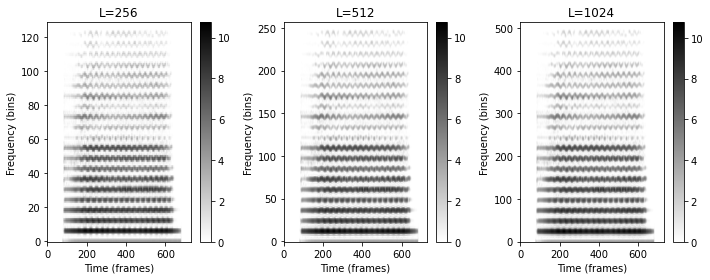Next, we repeat the same computation, where the axis are now converted to show physical units specified in seconds and Hertz. Furthermore, we zoom into the time-frequency plane to highlight the effect of having a denser frequency grid density.

In :
def plot_compute_spectrogram_physical(x, Fs, N, H, L, xlim, ylim, color='gray_r'):
Y, F_coef, T_coef = compute_stft(x, Fs, N, H, L)
extent=[T_coef, T_coef[-1], F_coef, F_coef[-1]]
plt.imshow(Y, cmap=color, aspect='auto', origin='lower', extent=extent)
plt.xlabel('Time (seconds)')
plt.ylabel('Frequency (Hz)')
plt.title('L=%d' % L)
plt.ylim(ylim)
plt.xlim(xlim)
plt.colorbar()

xlim_sec = [2, 3]
ylim_hz = [2000, 3000]

plt.figure(figsize=(10, 4))

L = N
plt.subplot(1,3,1)
plot_compute_spectrogram_physical(x, Fs, N, H, L, xlim=xlim_sec, ylim=ylim_hz)

L = 2 * N
plt.subplot(1,3,2)
plot_compute_spectrogram_physical(x, Fs, N, H, L, xlim=xlim_sec, ylim=ylim_hz)

L = 4 * N
plt.subplot(1,3,3)
plot_compute_spectrogram_physical(x, Fs, N, H, L, xlim=xlim_sec, ylim=ylim_hz)

plt.tight_layout()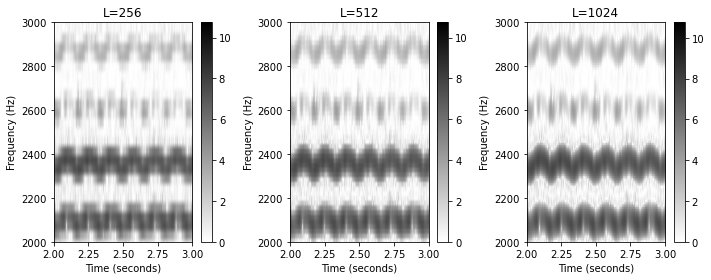## Further Notes¶

Acknowledgment: This notebook was created by Meinard Müller.